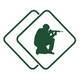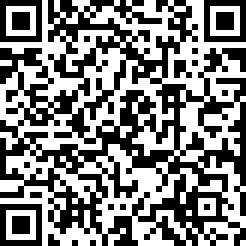#### ASVAB Armed Services Vocational Aptitude Battery (Exam 29)

created by Fisher BRink (@fisher) at Feb. 8, 2016
• The reciprocal of 1/6 is:

• If 0.05 ÷ x = 1, then x =

• Factor x2 6x + 9.

• (3 × 2)(7 2)(6 + 2) = (6 × 4)x. What's the value of x?

• Solve for x: 2x 6 = x + 5

• If I = prt, and p = \$1,000, r = 7%, and t = 1,what does I equal?

• x + y = 6 and x y = 4. Solve for x.

• Solve for y: 4(y + 3) + 7 = 3

• 12 yards + 14 feet ÷ by 2 =

• x3 × x3 =

• 41/5 + 12/5 + 33/10 =

• 5 × 102 =

• (900 × 2) ÷ 6 =

• The cube root of 64 is:

• A circle has a radius of 5 inches. What's its approximate area?

• Solve the following inequity:2/3(6x 9) + 4 &gt; 5x + 1

• A tube has a radius of 3 inches and a height of 5 inches. What's its approximate v...

• Triangle ABC (shown above) is a(n):

• The figure above is what type of quadrilateral?

• Angle AB (shown above) is a(n):

Be the first to review

• info
Quiz Info
• date_range
Feb. 8, 2016, 3:26 a.m.
help_outline
20 questions
dvr
0 completed
remove_red_eye
11 views
people
0 takers
folder•#### Ratings

star_borderstar_borderstar_borderstar_borderstar_border
ratings